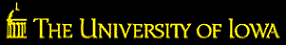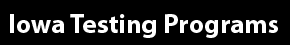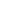Our sites: Primary Website | Login to Online Tools | Login to Bar Coding | Login to eITP# IEOC Geometry Content Coverage

The Iowa End-of-Course assessments are designed to assess student knowledge and skills in a variety of subject areas. The test consists of 30 multiple-choice items and is designed to be administered during a single class period. The test items, which are aligned to the Common Core State Standards, reflect educator input and a rigorous quality-control process to ensure appropriateness, content accuracy, and clarity.

A summary of the content covered within the Geometry test is presented below.

## Functions Interpreting Functions

Topics may include using function notation and analyzing linear, quadratic, square root, cube root, piecewise-defined, polynomial, rational, exponential, and logarithmic functions.

## Geometry Congruence

Topics may include representing and analyzing transformations in the coordinate plane; understanding congruence in terms of rigid motions; proving geometric theorems, including theorems about lines and angles, triangles, and parallelograms; and making geometric constructions.

## Similarity, Right Triangles, and Trigonometry

Topics may include understanding similarity in terms of similarity transformations, using congruence and similarity criteria to solve problems and prove relationships in geometric figures, defining trigonometric ratios and solving problems involving right triangles, and applying trigonometry to general triangles.

## Circles

Topics may include understanding and applying theorems about circles and finding arc lengths and areas of sectors of circles.

## Geometric Measurement and Dimension

Topics may include understanding the origins of formulas, including circumference and area of a circle and volumes of cylinders, pyramids, cones, and spheres; using formulas to solve problems; and visualizing relationships between two-dimensional and three-dimensional objects.

## Modeling with Geometry

Topics may include applying geometric concepts to model real-world phenomena.